Next: Optional additional lines in Up: The file `mcfgen.input' Previous: The file `mcfgen.input'   Contents

## Structure of the file `mcfgen.input'

The following is a typical first line of the file `mcfgen.input',

```     0  1  1  6  1 33  2     0  0                 00
```
(In practice several of the zeros are usually replaced by spaces, but these spaces are read as zeros.) The spacing is rigidly prescribed by the format,

READ(iocins,'(i6,1x,6(i2,1x),3x,i2, i3, 17x, 2i1)', & iostat=istat) & nnzab, iyi, iyo, ngpi, nflx, nppbe, ntmp, & idum, nclibtyp, nfiss, nsup

The meanings of these values is as follows.

nnzab:
The atomic number and mass number ( 1000*Z + A) for this target. The value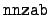= 0 is most common, and it serves as a flag indicating that nnzab is overwritten by the next line in the `mcfgen.input' file. A value of A = 0 denotes the natural element, so that ZA = 28000 represents natural nickel. The special values ZA = 99120 and 99125 denote, respectively, short-lived and long-lived fission fragments.

iyi:
The identifier for the incident particle. See Section 2.1. In the example above the `1' denotes a neutron.

iyo:
The identifier for the secondary particle. In the example line we have a neutron,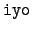= 1. This parameter is not used.

ngpi:
If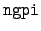> 0, it identifies which energy group bounds are to be read from the `bdfls' file. In the instance above we have= 6, so we take the collection of energy groups labeled `6' (175 groups with 20 Mev maximum). Note that for incident charged particles we usually have= 71 giving 63 energy groups with 20 Mev maximum, and for incident photons the standard value is= 80 for 175 groups with 30 Mev maximum. In order to specify the energy group boundaries from the `mcfgen.input' file, one sets= 0.

nflx:
A flux identifier. This is the flag specifying flux data in the `bdfls' file. The value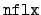= 1 gets an energy-independent flux. In order to get a non-constant flux, one may specify one of the other flux identifiers in the `bdfls' file, or one may set= 0, denoting that flux is given in the `mcfgen.input' file.

nppbe:
The number of equiprobable angular bin boundaries (33 here) for data type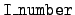= 3. This is reset to 17 if it is 0 here. The number of equiprobable angular bins is one less.

ntmp:
The temperature identifier. Usually,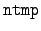> 0 and it is the flag for temperature data in the `bdfls' file. In the example it is= 2, giving the set `2' of temperatures. If= 0, we get the temperatures from the `mcfgen.input' file. In fact, there is to be only one temperature because Doppler broadening is not done within mcfgen.

idum:
Unused (an old debug option).

nclibtyp:
Defines the data type: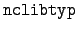= 0:
The output is in terms of bin averages.= 1:
The output is grid based. This option is used only for incident photons.

nfiss:
Not used by mcfgen.

nsup:
The temperature or level range request sentinel.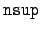= 0:
No special excitation levels or range of temperatures. This is the usual choice.= 1:
Read in the range of excitation levels from the input file `mcfgen.input'.= 2:
Get the range of temperatures from the `mcfgen.input' file. But note again that there can be only one temperature because mcfgen does not handle Doppler broadening.= 3:
Read in the ranges of excitation levels and temperatures from the `mcfgen.input' file.Next: Optional additional lines in Up: The file `mcfgen.input' Previous: The file `mcfgen.input'   Contents